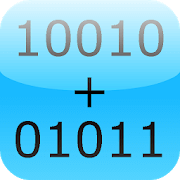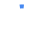ExpiredBinary Calculator Pro

\$0.99 FREE

This app is the pro version of Binary Calculator. Completely without advertisement!

This is a free mathematical calculator, which is able to add, subtract, multiply and divide numbers in binary format (used in computers).

Any number can be represented by any sequence of bits (binary digits), they are commonly written using the symbols 0 and 1.

Best math tool for school and college! If you are a student, it will helps you to learn arithmetics for computer science and electrical engineering.

Thanks for voting !!
 100% 0%Subscribe To Get All Offers In Your Inbox Every Day